### LIMITS OF FUNCTIONS AS X APPROACHES A CONSTANT

The following problems require the use of the algebraic computation of limits of functions as x approaches a constant. Most problems are average. A few are somewhat challenging. All of the solutions are given WITHOUT the use of L'Hopital's Rule. If you are going to try these problems before looking at the solutions, you can avoid common mistakes by giving careful consideration to the formduring the computations of these limits. Initially, many students INCORRECTLY conclude thatis equal to 1 or 0 , or that the limit does not exist or isor. In fact, the formis an example of an indeterminate form. This simply means that you have not yet determined an answer. Usually, this indeterminate form can be circumvented by using algebraic manipulation. Such tools as algebraic simplification, factoring, and conjugates can easily be used to circumvent the formso that the limit can be calculated.
• PROBLEM 1 : Compute.

• PROBLEM 2 : Compute.

• PROBLEM 3 : Compute.

• PROBLEM 4 : Compute.

• PROBLEM 5 : Compute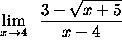.

• PROBLEM 6 : Compute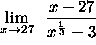.

• PROBLEM 7 : Compute.

• PROBLEM 8 : Compute.

• PROBLEM 9 : Compute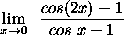.

• PROBLEM 10 : Compute.

• PROBLEM 11 : Compute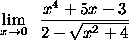.

• PROBLEM 12 : Compute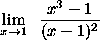.

• PROBLEM 13 : Compute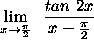.

This problem requires an unusual replacement, trigonometry identities, and trigonometry limits.

### The next problem requires an understanding of one-sided limits.

• PROBLEM 14 : Consider the functioni.) Sketch the graph of f .

ii.) Determine the following limits.

• a.)• b.)• c.)• d.)• e.)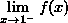• f.)• g.)• h.)• i.)• j.)• k.)• l.)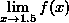• PROBLEM 15 : Consider the function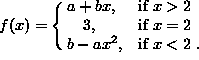Determine the values of constants a and b so thatexists and is equal to f(2).